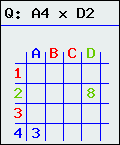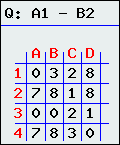# HelpAbove the grid is a question. In the grid there are 16 numbers (0-9) which are generated inside it. What you have to do is work out the answer of the mathematical equation. Here are some examples:

• Subtract: 9 - 4 = 5
• Addition: 9 + 4 = 13
• Multiplication: 9 x 4 = 36

For the example (on the left) we need to work out what A4 x D2 is. Looking at the grid (on the left) you can see that A4=3 and D2=8. When you multiply these two numbers you get 24.Once the username, password and answer are correct, you can successfully login. If you submit any incorrect login details you will see an error message. There are number of attempts (currently 10) by the time you are locked out (so be warned before contacting the administrator). If no attempts are made for five minutes, you will be allowed to login again.

If you find anything in the grid hard to see, please click the image to generate a new image (image on right).

If you find the grid hard to read or have any difficulties with the maths, please see the accessibility section.